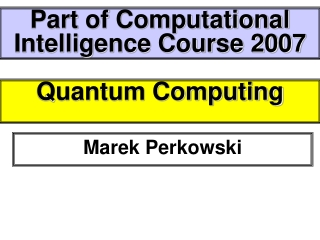DownloadDownload PresentationQuantum Computing

# Quantum Computing

Télécharger la présentation## Quantum Computing

- - - - - - - - - - - - - - - - - - - - - - - - - - - E N D - - - - - - - - - - - - - - - - - - - - - - - - - - -
##### Presentation Transcript

1. Part of Computational Intelligence Course 2007 Quantum Computing Marek Perkowski

2. Introduction to Quantum Logic and Reversible/Quantum Circuits Marek Perkowski

3. Historical Background and Links Quantum Computation & Quantum Information Study of information processing tasks that can be accomplished using quantum mechanical systems Cryptography Quantum Mechanics Information Theory Computer Science Digital Design

4. What is quantum computation? Computation with coherentatomic-scale dynamics. The behavior of a quantum computer is governed by the laws of quantum mechanics.

5. Why bother with quantum computation? Moore’s Law: We hit the quantum level 2010~2020. Quantum computation is more powerful than classical computation. More can be computed in less time—the complexity classes are different!

6. The power of quantum computation In quantum systems possibilities count, even if they never happen! Each of exponentially many possibilities can be used to perform a part of a computation at the same time.

7. Nobody understands quantum mechanics “No, you’re not going to be able to understand it. . . . You see, my physics students don’t understand it either. That is because I don’t understand it. Nobody does. ... The theory of quantum electrodynamics describes Nature as absurd from the point of view of common sense. And it agrees fully with an experiment. So I hope that you can accept Nature as She is -- absurd. Richard Feynman

8. Absurd but taken seriously (not just quantum mechanics but also quantum computation) Under active investigation by many of the top physics labs around the world (including CalTech, MIT, AT&T, Stanford, Los Alamos, UCLA, Oxford, l’Université de Montréal, University of Innsbruck, IBM Research . . .) In the mass media (including The New York Times, The Economist, American Scientist, Scientific American, . . .) Here.

9. Quantum Logic Circuits

10. A beam splitter Half of the photons leaving the light source arrive at detector A; the other half arrive at detector B.

11. A beam-splitter The simplest explanation is that the beam-splitter acts as a classical coin-flip, randomly sending each photon one way or the other.

12. An interferometer Equal path lengths, rigid mirrors. Only one photon in the apparatus at a time. All photons leaving the source arrive at B. WHY?

13. Possibilities count There is a quantity that we’ll call the “amplitude” for each possible path that a photon can take. The amplitudes can interfereconstructively and destructively, even though each photon takes only one path. The amplitudes at detector A interfere destructively; those at detector B interfere constructively.

14. Calculating interference Arrows for each possibility. Arrows rotate; speed depends on frequency. Arrows flip 180o at mirrors, rotate 90o counter-clockwise when reflected from beam splitters. Add arrows and square the length of the result to determine the probability for any possibility.

15. Double slit interference

16. Quantum Interference : Amplitudes are added and not intensities !

17. Interference in the interferometer Arrows flip 180o at mirrors, rotate 90o counter-clockwise when reflected from beam splitters

18. Quantum Interference Two beam-splitters The simplest explanation must be wrong, since it would predict a 50-50 distribution. How to create a mathematical model that would explain the previous slide and also help to predict new phenomena?

19. More experimental data

20. A new theory The particle can exist in a linear combination or superposition of the two paths

21. Probability Amplitude and Measurement If the photon is measured when it is in the state then we get with probability and |1> with probability of |a1|2

22. Quantum Operations The operations are induced by the apparatus linearly, that is, if and then

23. Quantum Operations Any linear operation that takes states satisfying and maps them to states satisfying must be UNITARY

24. Linear Algebra is unitary if and only if

25. Linear Algebra corresponds to corresponds to corresponds to

26. Linear Algebra corresponds to corresponds to

27. Linear Algebra corresponds to

28. Abstraction The two position states of a photon in a Mach-Zehnder apparatus is just one example of a quantum bit or qubit Except when addressing a particular physical implementation, we will simply talk about “basis” states and and unitary operations like and

29. where corresponds to and corresponds to

30. An arrangement like is represented with a network like

31. More than one qubit If we concatenate two qubits we have a 2-qubit system with 4 basis states and we can also describe the state as or by the vector 1

32. More than one qubit In general we can have arbitrary superpositions where there is no factorizationinto the tensor product of two independent qubits. These states are called entangled.

33. Entanglement Qubits in a multi-qubit system are not independent—they can become “entangled.” To represent the state of n qubits we use 2n complex number amplitudes.

34. Measuring multi-qubit systems If we measure both bits of we get with probability

35. Measurement ||2, for amplitudes of all states matching an output bit-pattern, gives the probability that it will be read. Example: 0.316|00› + 0.447|01› + 0.548|10› + 0.632|11› The probability to read the rightmost bit as 0 is |0.316|2 + |0.548|2 = 0.4 Measurement during a computation changes the state of the system but can be used in some cases to increase efficiency (measure and halt or continue).

36. Sources Origin of slides: John Hayes, Peter Shor, Martin Lukac, Mikhail Pivtoraiko, Alan Mishchenko, Pawel Kerntopf, Mosca, Ekert Mosca, Hayes, Ekert, Lee Spector in collaboration with Herbert J. Bernstein, Howard Barnum, Nikhil Swamy {lspector, hbernstein, hbarnum, nikhil_swamy}@hampshire.edu} School of Cognitive Science, School of Natural Science Institute for Science and Interdisciplinary Studies (ISIS) Hampshire College